# 工作中可能会使用到的数据结构和算法前端工程师 @ 公众号：ELab团队

## 背景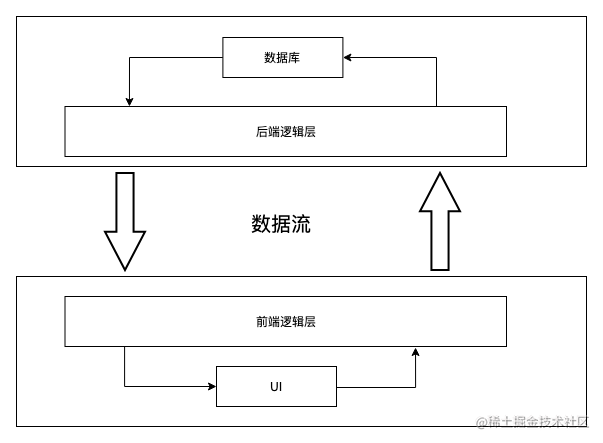## 数据结构

### 栈

##### 原理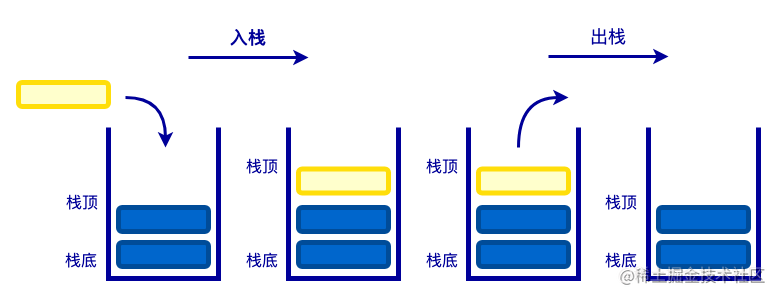##### 实现

``````class Stack {

constructor() {

// 存储数据

this.items = [];

}

push(item) {

// 入栈

this.items.push(item);

}

pop() {

// 出栈

return this.items.pop();

}

top() {

// 获取栈顶元素

return this.items[this.items.length - 1];

}

clear() {

// 清空栈

this.items = [];

}

size() {

// 获取栈的大小

return this.items.length;

}

isEmpty() {

// 判断栈是否为空

return this.items.length === 0;

}

}

##### 应用
1. 判断括号是否匹配

• 首先从头到尾遍历整个字符串；
• 当遇到字符"("则入栈，遇到字符")"则出栈；
• 出栈时，如果栈已经为空，则返回 false；
• 当字符串遍历完毕以后，判断栈是否为空。

• 声明变量 num 为 0，并从头到尾遍历整个字符串；
• 当遇到字符"("则 num 加 1，遇到字符")"num 减 1；
• 在遍历的过程中，当 num 减 1 时，num 的值已经为 0 则返回 false；
• 当字符串遍历完毕以后，判断 num 是否为 0。
``````// 方式一：栈

function isPairing(str = '') {

const stack = new Stack();

for(let i of str) {

if (i === '(') {

stack.push(i);

} else if (i === ')') {

if (stack.isEmpty()) {

return false;

} else {

stack.pop();

}

}

}

return stack.size() === 0;

}

// 方式二：计数

function isPairing(str = '') {

let num = 0;

for(let i of str) {

if (i === '(') {

num++;

} else if (i === ')') {

if (num === 0) {

return false;

} else {

num--;

}

}

}

return num === 0;

}

1. 判断 HTML 标签是否匹配

• 声明变量 stack、nodes；并从头遍历 HTML 字符串，查找字符"<"的位置；

• 如果字符"<"的位置等于 0：

• 则继续尝试匹配 HTML 结束标签，匹配成功并且与栈顶的标签名称一致，则弹出栈顶；否则报错；
• 匹配 HTML 结束标签失败以后，则尝试匹配开始标签的起始部分，然后循环匹配标签属性对，最后匹配开始标签的结束部分。匹配完成以后，将匹配到的标签压入栈顶；并构建 node 节点数；
• 如果字符"<"的位置大于 0：

• 则 html.slice(0, pos)，创建文本节点。
``````function parseHtml(html = '') {

const startIndex = 0;

const endIndex = 0;

// 匹配标签<div>、<br/>等标签的开始部分"<div、<br"

const startTagOpen = /^<([a-zA-Z\d]+)/;

// 匹配标签<div>、<br/>等标签的闭合部分">、/>"

const startTagClose = /^\s*(\/?)>/;

// 匹配属性

const attribute = /^\s*([\w-]+)(?:="([^"]*)")?\s*/;

// 匹配闭合标签，例如</div>、</p>

const endTag = /^<\/([a-zA-Z\d]+)>/;

const stack = [];

const nodes = [];

while(html) {

// 查找<的起始位置

const index = html.indexOf('<');

if (index === 0) {

// 先匹配整体结束标签，例如</div>、</p>

let endTagMatch = html.match(endTag);

if (endTagMatch) {

if (stack[stack.length - 1]) {

if (stack[stack.length - 1].tag === endTagMatch) {

// 出栈

stack.pop();

continue;

} else {

throw new Error(`起始标签和结束标签不匹配: 起始标签（\${stack[stack.length - 1].tag}），结束标签（\${endTagMatch}）`);

}

} else {

throw new Error(`\${endTagMatch}没有起始标签`);

}

}

// 然后匹配起始标签的开始部分，例如<div>的<div、<p>的<p、<br/>的<br

let startTagOpenMatch = html.match(startTagOpen);

if (startTagOpenMatch) {

const node = {

nodeType: 1,

tag: startTagOpenMatch,

attrs: [],

children: [],

};

let end, attr;

// 匹配标签属性列表

while(!(end = html.match(startTagClose)) && (attr = html.match(attribute))) {

node.attrs.push({

name: attr,

value: attr,

});

}

// 匹配起始标签的结束部分

if (end) {

if (stack.length === 0) {

nodes.push(node);

} else {

stack[stack.length - 1].children.push(node);

}

// 自闭和标签不加入栈中

if (end !== '/') {

stack.push(node);

}

}

}

} else {

// 文本

const node = {

nodeType: 3,

textContent: html.slice(0, index)

};

if (stack.length === 0) {

nodes.push(node);

} else {

stack[stack.length - 1].children.push(node);

}

}

}

html = html.substring(n);

}

return nodes;

}

parseHtml('<div id="test" class="a b"></div>');

parseHtml('<div id="test" class="a b">Hello World</div>');

parseHtml('开始<div id="test" class="a b">Hello World</div>');

parseHtml('<div id="test" class="a b"><br class="br" />Hello World</div>');

parseHtml('</div>');

parseHtml('<div></p>');

1. 版本号比较大小

• 版本号 v1、v2 按照符号"."分割成数组，从左右依次进行大小比较；
• v1 大于 v2 返回 1，v2 小于 v2 返回-1，v1 等于 v2 返回 0。
``````/*

比较版本号大小

v1：第一个版本号

v2：第二个版本号

如果版本号相等，返回 0, * 如果第一个版本号低于第二个，返回 -1，否则返回 1.

*/

function compareVersion(v1, v2) {

if (!v1 && !v2) return 0;

if (!v1) return -1;

if (!v2) return 1;

const v1Stack = v1.split('.');

const v2Stack = v2.split('.');

const maxLen = Math.max(v1Stack.length, v2Stack.length);

for(let i = 0; i < maxLen; i++) {

// 必须转整，否则按照字符顺序比较大小

const prevVal = ~~v1Stack[i];

const currVal = ~~v2Stack[i];

if (prevVal > currVal) {

return 1;

}

if (prevVal < currVal) {

return -1;

}

}

return 0;

}

console.log(compareVersion("2.2.1", "2.2.01")); // 0

console.log(compareVersion("2.2.1", "2.2.0")); // 1

console.log(compareVersion("2.2.1", "2.1.9")); // 1

console.log(compareVersion("2.2", "2.1.1")); // 1

console.log(compareVersion("2.2", "2.2.1")); // -1

console.log(compareVersion("2.2.3.4.5.6", "2.2.2.4.5.12")); // 1

console.log(compareVersion("2.2.3.4.5.6", "2.2.3.4.5.12")); // -1

##### 用途
• Vue 模板编译将模板字符串转换成 AST。
• 自动更新最新版本的 NPM 包。
• 函数执行上下文栈。

### 队列

##### 原理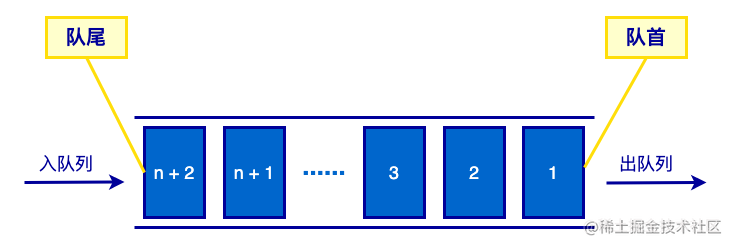##### 实现

``````class Queue {

constructor() {

// 存储数据

this.items = [];

}

enqueue(item) {

// 入队

this.items.push(item);

}

dequeue() {

// 出队

return this.items.shift();

}

// 获取队首的元素

return this.items;

}

tail() {

// 获取队尾的元素

return this.items[this.items.length - 1];

}

clear() {

// 清空队列

this.items = [];

}

size() {

// 获取队列的长度

return this.items.length;

}

isEmpty() {

// 判断队列是否为空

return this.items.length === 0;

}

}

##### 应用
1. 约瑟夫环问题

• 创建队列，将 0 到 99 的数字入队；
• 循环队列，依次出列队列中的数字，对当前出队的数字进行计数 index + 1；
• 判断当前出列的 index % 3 是否等于 0，如果不等于 0 则入队；
• 直到队列的长度为 1，退出循环，返回队列中的数字。
``````function ring(arr) {

const queue = new Queue();

arr.forEach(v => queue.enqueue(v));

let index = 0;

while(queue.size() > 1) {

const item = queue.dequeue();

if (++index % 3 !== 0) {

queue.enqueue(item);

}

}

}

1. 斐波那契数列

``````function fiboSequence(num) {

if (num < 2) return num;

const queue = [];

queue.push(0);

queue.push(1);

for(let i = 2; i < num; i++) {

const len = queue.length;

queue.push(queue[len - 2] + queue[len  - 1]);

}

return queue;

}

1. 打印杨辉三角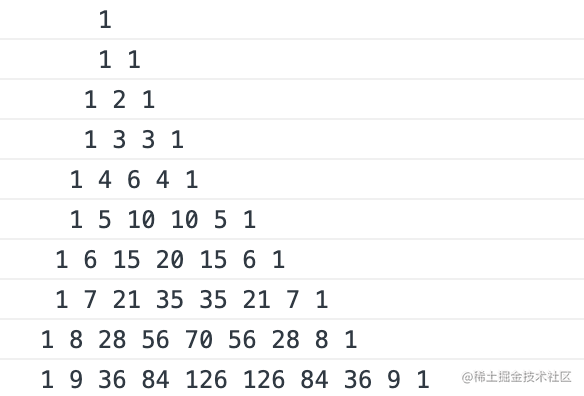• 通过观察发现，三角中的每一行数据都依赖于上一行的数据；
• 我们首先创建队列 queue，用于存储每一行的数据，供下一行数据使用；
• 然后初始化第一行的数据 1 入队，这里需要两个 for 循环嵌套，外层的 for 循环决定最终打印的总行数，内层的 for 循环生成每行的数据；
• 在生成当前行的数据时，将队列中的数据源依次出队，然后将新生成的数据入队；并记录当前出队的数据，供生成新数据使用。
``````function printYangHui(num) {

const queue = [];

// 存储第一行数据

queue.push(1);

for(let i = 1; i <= num; i++) {

let rowArr = [];

// 填充空格

for(let j = 0; j < Math.floor((num - i) / 2); j++) {

rowArr.push('');

}

let prev = 0;

for(let j = 0; j < i; j++) {

const num = queue.shift();

queue.push(prev + num);

rowArr.push(num);

prev = num;

}

queue.push(1);

console.log(rowArr.join(' '));

}

}

printYangHui(10);

##### 用途
1. 实现洋葱模型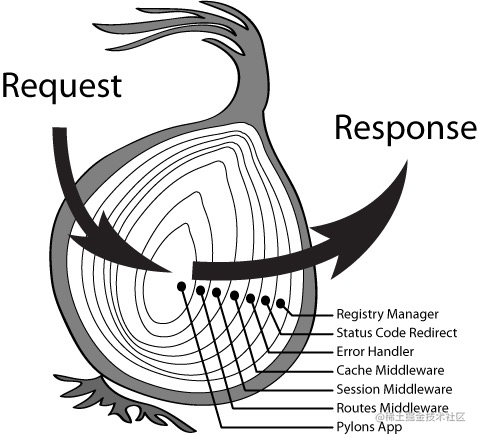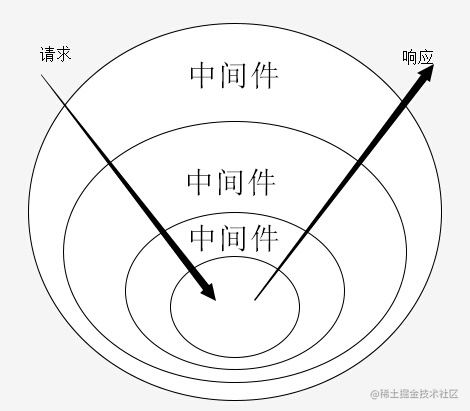``````function createApp(){

return {

use(fn){},

run(){},

}

}

const app = createApp();

app.use((next)=>{

setTimeout(function(){

next();

})

console.log(new Date() ,'1');

})

app.use((next)=>{

console.log(new Date() ,'2');

next();

})

app.use((next)=>{

console.log(new Date() ,'3');

next();

})

app.run();

1. 消息队列

### 链表

##### 分类
• 单向链表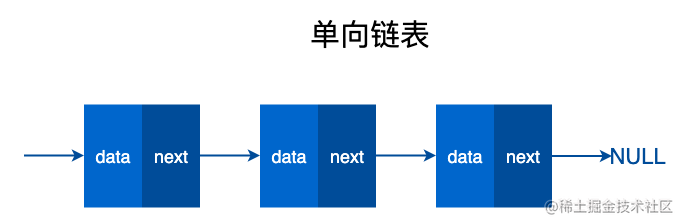• 双向链表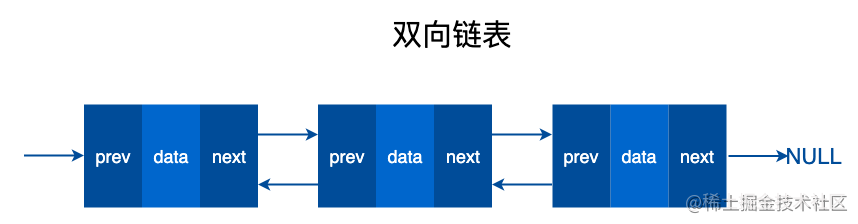• 单向循环链表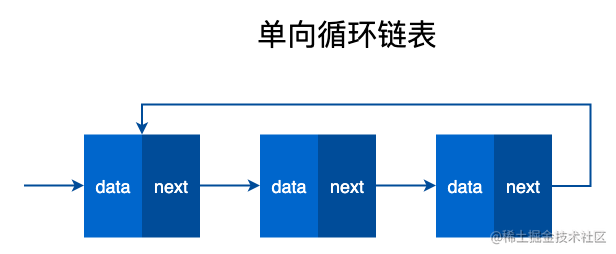• 双向循环链表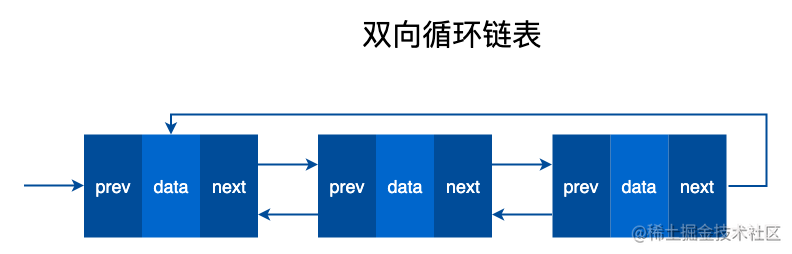##### 实现
``````const Node = function (data) {

this.data = data;

this.next = null;

}

const node1 = new Node(1);

const node2 = new Node(2);

const node3 = new Node(3);

node1.next = node2;

node2.next = node3;

##### 应用
1. 环形链表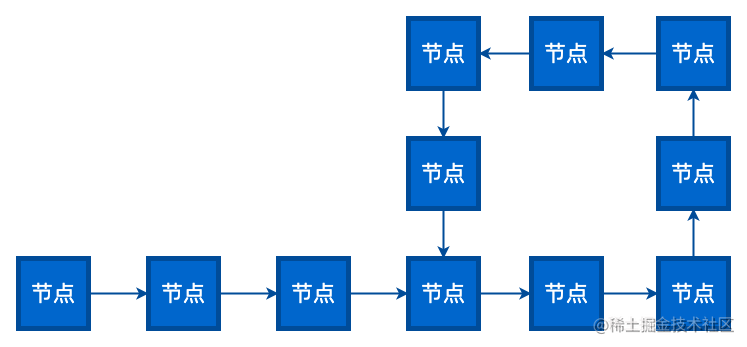1. 首先创建两个指针 1 和 2，同时指向这个链表的头节点。然后开始一个大循环，在循环体中，让指针 1 每次向下移动一个节点，让指针 2 每次向下移动两个节点，然后比较两个指针指向的节点是否相同。如果相同，则判断出链表有环，如果不同，则继续下一次循环。
2. 例如链表 A->B->C->D->B->C->D，两个指针最初都指向节点 A，进入第一轮循环，指针 1 移动到了节点 B，指针 2 移动到了 C。第二轮循环，指针 1 移动到了节点 C，指针 2 移动到了节点 B。第三轮循环，指针 1 移动到了节点 D，指针 2 移动到了节点 D，此时两指针指向同一节点，判断出链表有环。
3. 假设从链表头节点到入环点的距离是 D，链表的环长是 S。那么循环会进行 S 次，可以简单理解为 O（N）。除了两个指针以外，没有使用任何额外存储空间，所以空间复杂度是 O（1）。
``````const Node = function (data) {

this.data = data;

this.next = null;

}

const nodeA = new Node('A');

const nodeB = new Node('B');

const nodeC = new Node('C');

const nodeD = new Node('D');

const nodeE = new Node('E');

nodeA.next = nodeB;

nodeB.next = nodeC;

nodeC.next = nodeD;

nodeD.next = nodeE;

nodeE.next = nodeC;

return false;

}

do {

point1 = point1.next;

point2 = point2.next && point2.next.next;

} while(point1 && point2 && point1 !== point2);

if (point1 === point2) {

return true;

}

return false;

}

1. 相交链表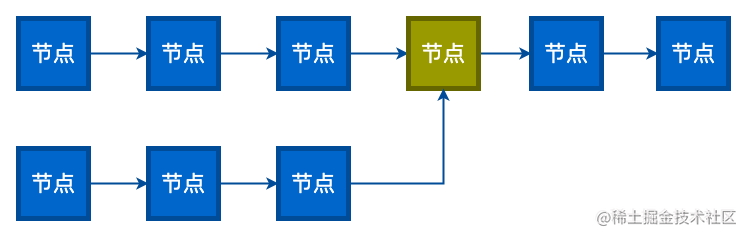1. 两个没有环的链表如果是相交于某一结点，如上图所示，这个结点后面都是共有的。所以如果两个链表相交，那么两个链表的尾结点的地址也是一样的。程序实现时分别遍历两个单链表，直到尾结点。判断尾结点地址是否相等即可。时间复杂度为 O(L1+L2)。
2. 如何找到第一个相交结点？判断是否相交的时候，记录下两个链表的长度，算出长度差 len，接着先让较长的链表遍历 len 个长度，然后两个链表同时遍历，判断是否相等，如果相等，就是第一个相交的结点。
``````function intersectNode(head1, head2) {

// 计算链表的长度

let len1 = 0, p = head1;

let len2 = 0, q = head2;

while(p.next) {

len1++;

p = p.next;

}

while(q.next) {

len2++;

q = q.next;

}

if (p === q) {

// p指向短链，q指向长链

let len = 0;

if (len1 > len2) {

len = len1 - len2;

} else {

len = len2 - len1;

}

while(len > 0) {

len--;

q = q.next;

}

while(p && q && p !== q) {

p = p.next;

q = q.next;

}

return p;

}

}

return null;

}

const Node = function (data) {

this.data = data;

this.next = null;

}

const nodeA = new Node('A');

const nodeB = new Node('B');

const nodeC = new Node('C');

const node1 = new Node('1');

const node2 = new Node('2');

const node3 = new Node('3');

const nodeD4 = new Node('D4');

const nodeE5 = new Node('E5');

nodeA.next = nodeB;

nodeB.next = nodeC;

nodeC.next = nodeD4;

node1.next = node2;

node2.next = node3;

node3.next = nodeD4;

nodeD4.next = nodeE5;

console.log(intersectNode(nodeA, node1));

1. 回文链表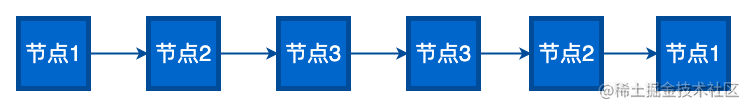1. 从头遍历链表，同时正向和反向拼接每个链表的数据，最后比对正向和反向得到的字符串是否相等。如果相等则是回文链表；否则不是。
``````const Node = function (data) {

this.data = data;

this.next = null;

}

const node1 = new Node('A');

const node2 = new Node('B');

const node3 = new Node('C');

const node4 = new Node('C');

const node5 = new Node('B');

const node6 = new Node('A');

node1.next = node2;

node2.next = node3;

node3.next = node4;

node4.next = node5;

node5.next = node6;

const isPalindrome = head => {

let a = '', b = '';

}

return a === b;

}

console.log(isPalindrome(node1));

1. 原型链
2. 作用域链

### 树

##### 分类
• 无序树：树中任意节点的子结点之间没有顺序关系，这种树称为无序树,也称为自由树。
• 有序树：树中任意节点的子结点之间有顺序关系，这种树称为有序树。
• 二叉树：每个节点最多含有两个子树的树称为二叉树。
• 满二叉树：叶节点除外的所有节点均含有两个子树的树被称为满二叉树。
• 完全二叉树：除最后一层外，所有层都是满节点，且最后一层缺右边连续节点的二叉树称为完全二叉树（堆就是一个完全二叉树）。
• 哈夫曼树（最优二叉树）：带权路径最短的二叉树称为哈夫曼树或最优二叉树。
##### 实现
``````// 二叉树的实现

function Node(data) {

this.data = data;

this.left = null;

this.right = null;

}

const nodeA = new Node('A');

const nodeB = new Node('B');

const nodeC = new Node('C');

const nodeD = new Node('D');

const nodeE = new Node('E');

const nodeF = new Node('F');

const nodeG = new Node('G');

nodeA.left = nodeB;

nodeA.right = nodeC;

nodeB.left = nodeD;

nodeB.right = nodeE;

nodeC.left = nodeF;

nodeC.right = nodeG;

##### 遍历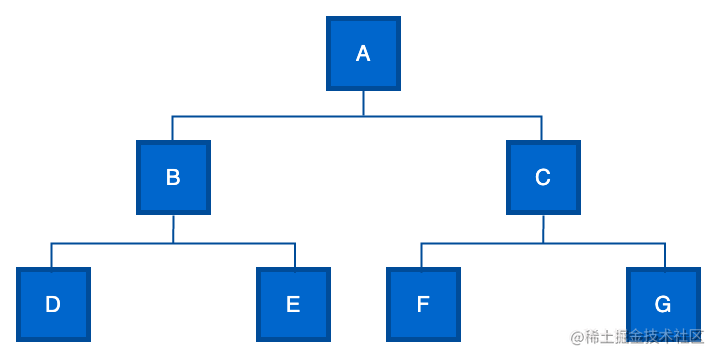• 深度优先遍历

• 先序遍历

• 中序遍历

• 后序遍历

• 广度优先遍历

• 层序遍历

##### 用途
1. 树的扁平化（展示 OCR 识别结果）
2. 扁平化数组转换成树（标签树）

### 图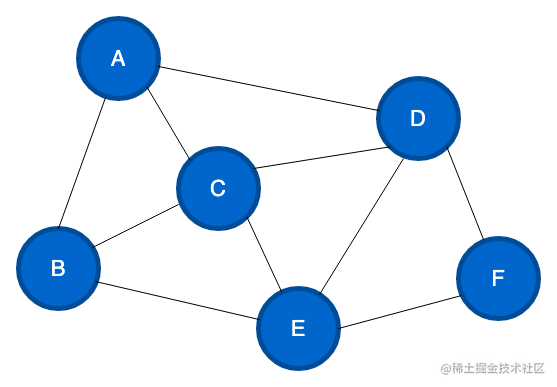• 无向图

• 有向图

• 加权图

• 连通图

##### 表示

• 邻接矩阵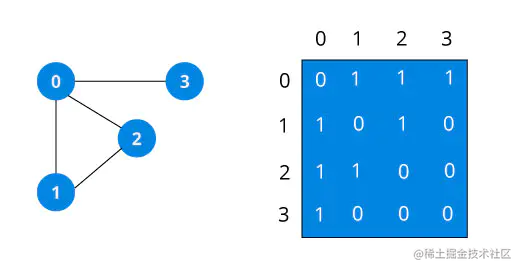• 邻接链表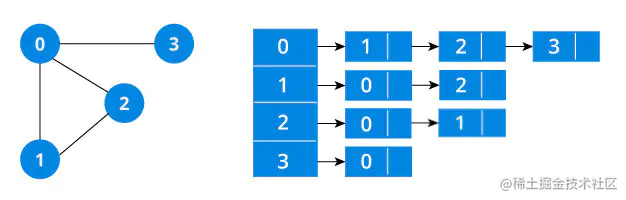• 深度优先遍历
• 广度优先遍历
##### 用途
• 商品分类选择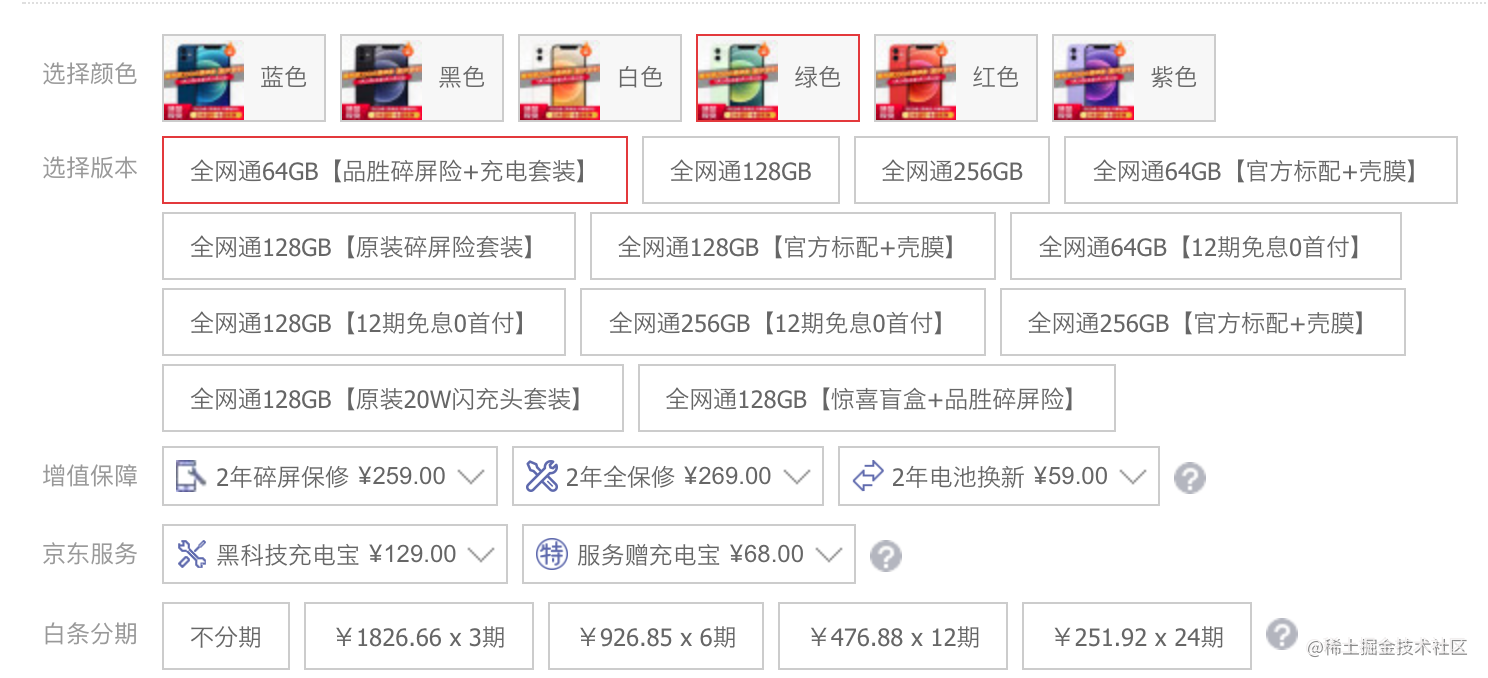## 算法

### LRU

LRU 是 Least Recently Used 的缩写，即最近最少使用，是一种常用的页面置换算法，将最近最久未使用的页面予以淘汰。

##### 原理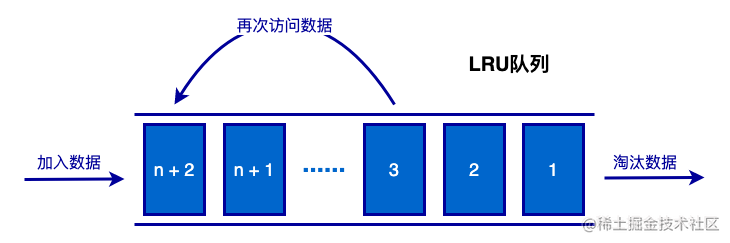##### 实现

• 选择合适的数据结构。

• 哈希表：O(1) 级别的时间复杂度，适合数据查找。但是元素无序，没办法判断元素访问的先后顺序。
• 数组：元素的插入和删除元素都是 O(n)。
• 单向链表：删除节点需要访问前驱节点，需要花 O(n)从前遍历查找。
• 双向链表：结点有前驱指针，删除和移动节点都是指针的变动，都是 O(1)。

• 设置虚拟节点，方便快速的访问头尾节点。初始时没有添加真实的节点，所以需要将虚拟节点的前驱指针和后继指针指向自己。

• get 方法的实现。

• put 方法的实现。

• 写入新数据，需要先检查一下当前节点数量；如果节点数量达到容量的最大值，则需要先删除链表尾部的节点，然后创建新的节点，添加到链表头部，并写入到哈希表。
• 写入已存在的数据，则更新数据值，移动节点位置到链表头部。
``````function Node(key, value) {

this.key = key;

this.value = value;

this.prev = null;

this.next = null;

}

class LRUCache {

constructor(capacity) {

this.capacity = capacity; // 容量

this.hash = {}; // 哈希表

this.count = 0; // 当前节点数量

this.virtualNode = new Node(); // 虚拟结点

// 相互引用

this.virtualNode.next = this.virtualNode;

this.virtualNode.prev = this.virtualNode;

}

get(key) {

const node = this.hash[key];

if (node) {

return node.value;

}

}

put(key, value) {

const node = this.hash[key];

if (node) {

node.value = value;

} else {

if (this.count === this.capacity) {

this.removeLRUItem();

}

const newNode = new Node(key, value);

this.hash[key] = newNode;

this.count++;

}

}

remove(key) {

const node = this.hash[key];

if (node) {

this.removeFromList(node);

Reflect.deleteProperty(this.hash, key);

this.count--;

}

}

isEmpty() {

return this.count === 0;

}

this.removeFromList(node);

}

removeFromList(node) {

const prevNode = node.prev;

const nextNode = node.next;

prevNode.next = nextNode;

nextNode.prev = prevNode;

node.prev = null;

node.next = null;

}

const nextNode = this.virtualNode.next;

this.virtualNode.next = node;

nextNode.prev = node;

node.prev = this.virtualNode;

node.next = nextNode;

}

removeLRUItem() {

const tailNode = this.virtualNode.prev;

this.remove(tailNode.key);

}

}

const cache = new LRUCache(5);

console.log(cache.isEmpty());

cache.put('A', 'A');

cache.put('B', 'B');

cache.put('C', 'C');

cache.put('D', 'D');

cache.put('E', 'E');

console.log(cache.get('A'));

cache.put('F', 'F');

console.log(cache.get('B'));

console.log(cache.isEmpty());

cache.remove('E');

cache.remove('F');

cache.remove('A');

cache.remove('C');

cache.remove('D');

console.log(cache.isEmpty());

console.log(cache);

• 历史浏览记录。
• 缓存淘汰策略。